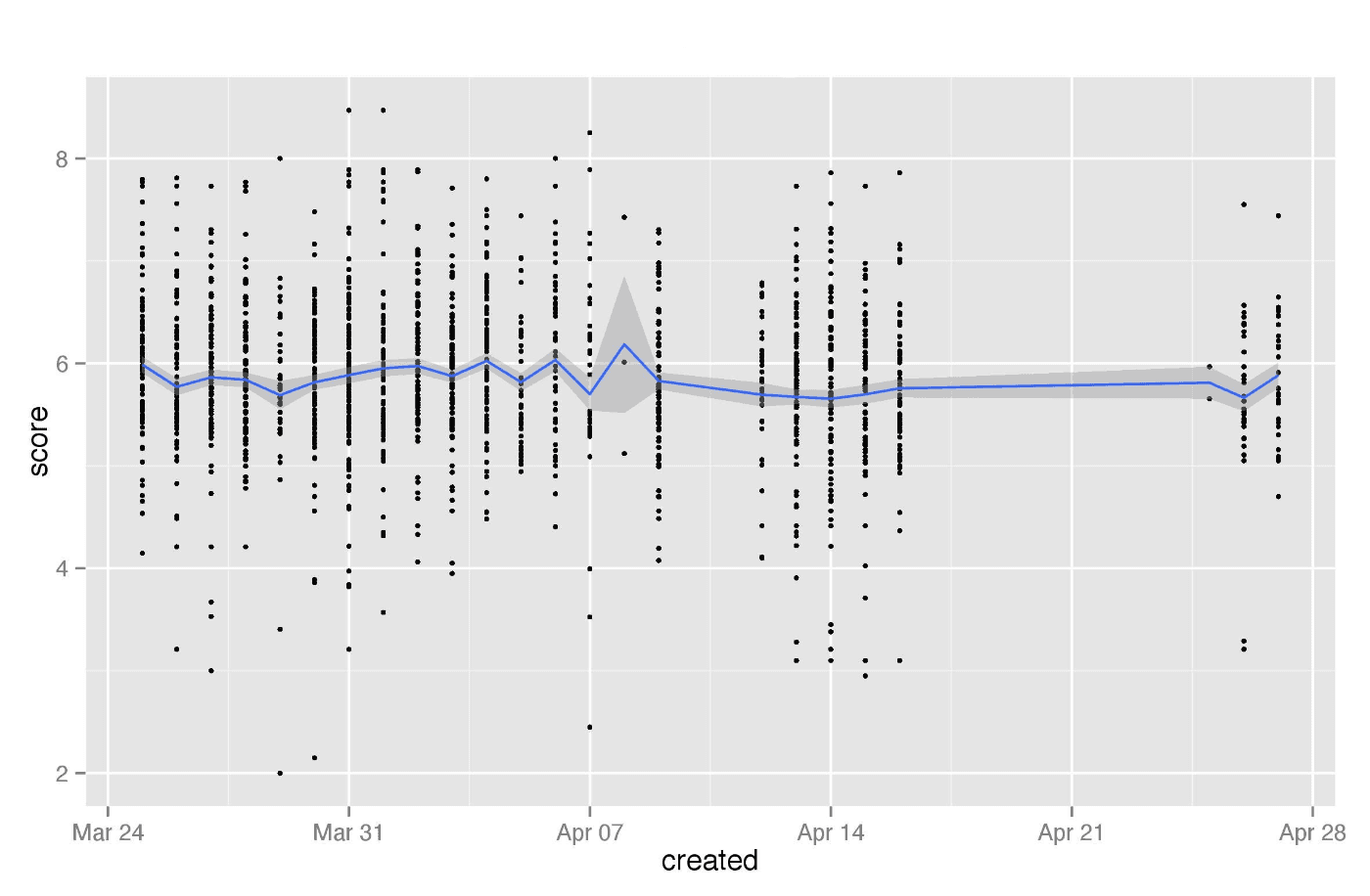Back to Home

Twitter sentiment analysis based on affective lexicons with R

Continue to dig tweets. After we reviewed how to count positive, negative and neutral tweets in the previous post, I discovered another great idea. Suppose positive or negative mark is not enough and we want to understand the rate of positivity or negativity.

For example, if word “good” has 4 points rating, but “perfect” has 6. In this way we can try to measure the rate of satisfaction or opinion in tweets and take a chart with the trend as the following:We need another dictionary for managing this task, specifically the dictionary with a rating of words. We can create it or find results of great research of affective ratings (e.g. here).

And of course, our algorithm should bypass Twitter’s API limitation via accumulating historical data. This approach was described in the previous post.

Note, I will use average rating for evaluating tweets based on words rating it consists of. For example, if we’ve found “good” (4 points) and “perfect” (6 points) in the tweet, it would be evaluated as (4+6)/2=5. In this way, we will avoid the influence of several negative words that could have a higher total rating, e.g. one word “good” (4 points) should have a higher rating than three words “bad” (for 1,5 points each).

Let’s start. We need to create Twitter Application (https://apps.twitter.com/) in order to have an access to Twitter’s API. Then we will get Consumer Key and Consumer Secret. And finally, our code in R:

```#connect all libraries
library(ROAuth)
library(plyr)
library(dplyr)
library(stringr)
library(ggplot2)```
```#connect to API
consumerKey <- '____________' #put the Consumer Key from Twitter Application
consumerSecret <- '______________'  #put the Consumer Secret from Twitter Application
Cred <- OAuthFactory\$new(consumerKey=consumerKey,
consumerSecret=consumerSecret,
requestURL=reqURL,
accessURL=accessURL,
authURL=authURL)
Cred\$handshake(cainfo = system.file('CurlSSL', 'cacert.pem', package = 'RCurl')) #There is URL in Console. You need to go to, get code and enter it on Console```
```save(Cred, file='twitter authentication.Rdata')
load('twitter authentication.Rdata') #Once you launched the code first time, you can start from this line in the future (libraries should be connected)
```#the function for extracting and analyzing tweets
search <- function(searchterm)
{
#extract tweets and create storage file
df <- twListToDF(list)
df <- df[, order(names(df))]
df\$created <- strftime(df\$created, '%Y-%m-%d')
if (file.exists(paste(searchterm, '_stack_val.csv'))==FALSE) write.csv(df, file=paste(searchterm, '_stack_val.csv'), row.names=F)```
```#merge the last extraction with storage file and remove duplicates
stack <- rbind(stack, df)
stack <- subset(stack, !duplicated(stack\$text))
write.csv(stack, file=paste(searchterm, '_stack_val.csv'), row.names=F)```
```#tweets evaluation function
score.sentiment <- function(sentences, valence, .progress='none')
{
require(plyr)
require(stringr)
scores <- laply(sentences, function(sentence, valence){
sentence <- gsub('[[:punct:]]', '', sentence) #cleaning tweets
sentence <- gsub('[[:cntrl:]]', '', sentence) #cleaning tweets
sentence <- gsub('\\d+', '', sentence) #cleaning tweets
sentence <- tolower(sentence) #cleaning tweets
word.list <- str_split(sentence, '\\s+') #separating words
words <- unlist(word.list)
val.matches <- match(words, valence\$Word) #find words from tweet in "Word" column of dictionary
val.match <- valence\$Rating[val.matches] #evaluating words which were found (suppose rating is in "Rating" column of dictionary).
val.match <- na.omit(val.match)
val.match <- as.numeric(val.match)
score <- sum(val.match)/length(val.match) #rating of tweet (average value of evaluated words)
return(score)
}, valence, .progress=.progress)
scores.df <- data.frame(score=scores, text=sentences) #save results to the data frame
return(scores.df)
}```
`valence <- read.csv('dictionary.csv', sep=',' , header=TRUE) #load dictionary from .csv file`
```Dataset <- stack
Dataset\$text <- as.factor(Dataset\$text)
scores <- score.sentiment(Dataset\$text, valence, .progress='text') #start score function
write.csv(scores, file=paste(searchterm, '_scores_val.csv'), row.names=TRUE) #save evaluation results into the file```
```#modify evaluation
stat <- scores
stat\$created <- stack\$created
stat\$created <- as.Date(stat\$created)
stat <- na.omit(stat) #delete unvalued tweets
write.csv(stat, file=paste(searchterm, '_opin_val.csv'), row.names=TRUE)```
```#chart
ggplot(stat, aes(created, score)) + geom_point(size=1) +
stat_summary(fun.data = 'mean_cl_normal', mult = 1, geom = 'smooth') +
ggtitle(searchterm)```
```ggsave(file=paste(searchterm, '_plot_val.jpeg'))
}```
`search("______") #enter keyword`

Finally, we will get 4 files:

• storage file with initial data,
• file with tweets rating,
• cleaned (without unvalued tweets) file with tweets and dates,
• the chart where we can see the density of tweet ratings and mean as a trend that looks like: